International
Tables for
Crystallography
Volume D
Physical properties of crystals
Edited by A. Authier

International Tables for Crystallography (2006). Vol. D, ch. 1.6, pp. 173-176

## Section 1.6.7. The linear photoelastic effect

A. M. Glazera* and K. G. Coxb

aDepartment of Physics, University of Oxford, Parks Roads, Oxford OX1 3PU, England, and bDepartment of Earth Sciences, University of Oxford, Parks Roads, Oxford OX1 3PR, England
Correspondence e-mail:  glazer@physics.ox.ac.uk

### 1.6.7. The linear photoelastic effect

| top | pdf |

#### 1.6.7.1. Introduction

| top | pdf |

The linear photoelastic (or piezo-optic) effect (Narasimhamurty, 1981) is given by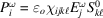, and, like the electro-optic effect, it can be discussed in terms of the change in dielectric impermeability caused by a static (or low-frequency) field, in this case a stress, applied to the crystal. This can be written in the form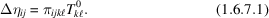The coefficientsform a fourth-rank tensor known as the linear piezo-optic tensor. Typically, the piezo-optic coefficients are of the order of 10−12 m2 N−1. It is, however, more usual to express the effect as an elasto-optic effect by making use of the relationship between stress and strain (see Section 1.3.3.2), thus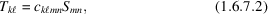where the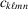are the elastic stiffness coefficients. Therefore equation (1.6.7.2)can be rewritten in the form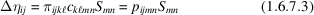or, in contracted notation,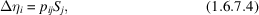where, for convenience, the superscript 0 has been dropped, the elastic strain being considered as essentially static or of low frequency compared with the natural mechanical resonances of the crystal. The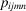are coefficients that form the linear elasto-optic (or strain-optic) tensor (Table 1.6.7.1). Note that these coefficients are dimensionless, and typically of order 10−1, showing that the change to the optical indicatrix is roughly one-tenth of the strain.

 Table 1.6.7.1| top | pdf | Symmetry constraints on the linear elasto-optic (strain-optic) tensor(contracted notation) (see Section 1.1.4.10.6)
TriclinicOrthorhombicTetragonalTrigonal
Point group 1 Point groups 222, mm2, mmm Point groups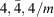Point groups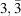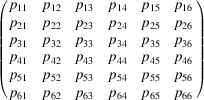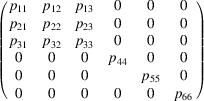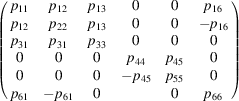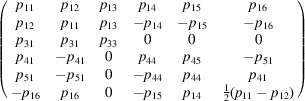Monoclinic Point groups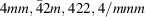Point groups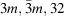Point groups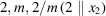Point groups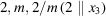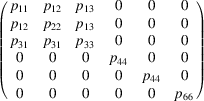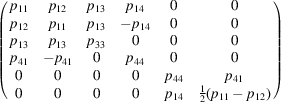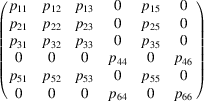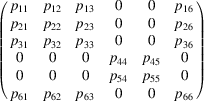HexagonalCubicIsotropic
Point groups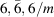Point groups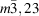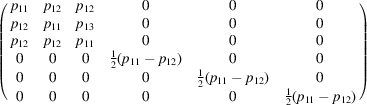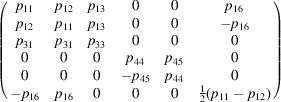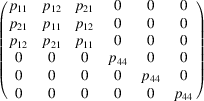Point groups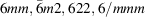Point groups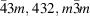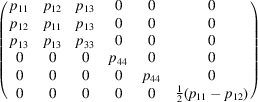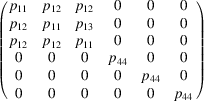The elasto-optic effect can arise in several ways. The most obvious way is through application of an external stress, applied to the surfaces of the crystal. However, strains, and hence changes to the refractive indices, can arise in a crystal through other ways that are less obvious. Thus, it is a common finding that crystals can be twinned, and thus the boundary between twin domains, which corresponds to a mismatch between the crystal structures either side of the domain boundary, will exhibit a strain. Such a crystal, when viewed between crossed polars under a microscope will produce birefringence colours that will highlight the contrast between the domains. This is known as strain birefringence. Similarly, when a crystal undergoes a phase transition involving a change in crystal system, a so-called ferroelastic transition, there will be a change in strain owing to the difference in unit-cell shapes. Hence there will be a corresponding change in the optical indicatrix. Often the phase transition is one going from a high-temperature optically isotropic section to a low-temperature optically anisotropic section. In this case, the high-temperature section has no internal strain, but the low-temperature phase acquires a strain, which is often called the spontaneous strain (by analogy with the term spontaneous polarization in ferroelectrics).

#### 1.6.7.2. Spontaneous strain in BaTiO3

| top | pdf |

As an example of the calculation of the relationship between spontaneous strain and linear birefringence, consider the high-temperature phase transition of the well known perovskite BaTiO3. This substance undergoes a transition at around 403 K on cooling from its high-temperature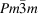phase to the room-temperaturephase. Thephase is both ferroelectric and ferroelastic. In this tetragonal phase, there is a small distortion of the unit cell along  and a contraction alongcompared with the unit cell of the high-temperature cubic phase, and so the room-temperature phase can be expected to have a uniaxial optical indicatrix.

The elasto-optic tensor for thephase is (Table 1.6.7.1)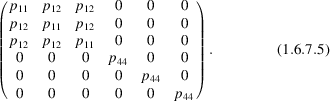Consider the low-temperature tetragonal phase to arise as a small distortion of this cubic phase, with a spontaneous strain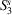given by the lattice parameters of the tetragonal phase: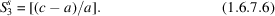Therefore, the equations (1.6.7.4)for the dielectric impermeability in terms of the spontaneous strain component are given in matrix form as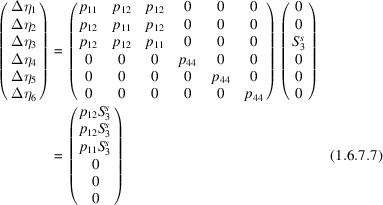so that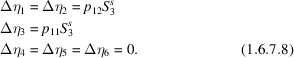By analogy with equations (1.6.6.5)and (1.6.6.6), the induced changes in refractive index are then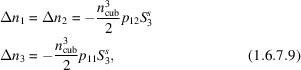where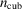is the refractive index of the cubic phase. Thus the birefringence in the tetragonal phase as seen by light travelling alongis given by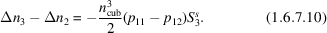Thus a direct connection is made between the birefringence of the tetragonal phase of BaTiO3 and its lattice parameters via the spontaneous strain. As in the case of the linear electro-optic effect, the calculation can be repeated using equation (1.6.3.14)with the susceptibilities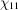and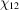to yield the relationship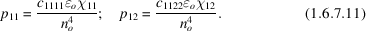#### 1.6.7.3. The acousto-optic effect

| top | pdf |

The acousto-optic effect (Sapriel, 1976) is really a variant of the elasto-optic effect, in that the strain field is created by the passage of a sound wave through the crystal. If this wave has frequency, the resulting polarization in the presence of a light wave of frequencyis given by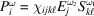, where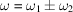. However, since the sound-wave frequency is very small compared with that of the light, to all intents and purposes the change in frequency of the light field can be ignored. The effect then of the sound wave is to produce within an acousto-optic crystal a spatially modulated change in refractive index: a beam of light can then be diffracted by this spatial modulation, the resulting optical diffraction pattern thus changing with the changing sound signal. Acousto-optic materials therefore can be used as transducers for converting sound signals into optical signals for transmission down optical fibres in communications systems. Consider, for instance, a sound wave propagating along the  direction in gallium arsenide (GaAs), which crystallizes in point group. Suppose that this sound wave is longitudinally polarized. With respect to the cube axes, this corresponds to an oscillatory shear strain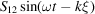, whereis a distance along the  direction (Fig. 1.6.7.1). Then one can write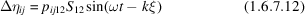or in contracted notation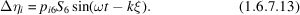From Table 1.6.7.1, it seen that the change in dielectric impermeability tensor is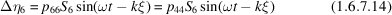since all other components are zero. This means that the original spherical indicatrix of the cubic crystal has been distorted to form a biaxial indicatrix whose axes oscillate in length according to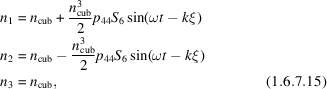thus forming an optical grating of spatial periodicity given by the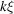term. In gallium arsenide, at a wavelength of light equal to 1.15 µm,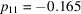,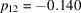and. It is convenient to define a figure of merit for acousto-optic materials (Yariv & Yeh, 1983) given by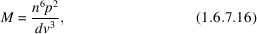where v is the velocity of the sound wave and d is the density of the solid. For gallium arsenide, d = 5340 kg m−3, and for a sound wave propagating as above v = 5.15 m s−1. At the wavelength λ= 1.15 µm, n = 3.37, and so it is found that M = 104. In practice, figures of merits can range from less than 0.001 up to as high as 4400 in the case of Te, and so the value for gallium arsenide makes it potentially useful as an acousto-optic material for infrared signals.Figure 1.6.7.1 | top | pdf |(a) Symmetry elements of point group. (b) Symmetry elements after strain applied along . (c) Effect on spherical indicatrix.

### References

Narasimhamurty, T. S. (1981). Photoelastic and electro-optic properties of crystals. New York: Plenum.
Sapriel, J. (1976). Acousto-optics. Chichester: Wiley.
Yariv, A. & Yeh, P. (1983). Optical waves in crystals. New York: Wiley.